# Formula Editor

Many of the ADL blocks allow you to define formulas to calculate output values for the blocks. For such blocks, you can open the Formula Editor from their respective Block Properties panel.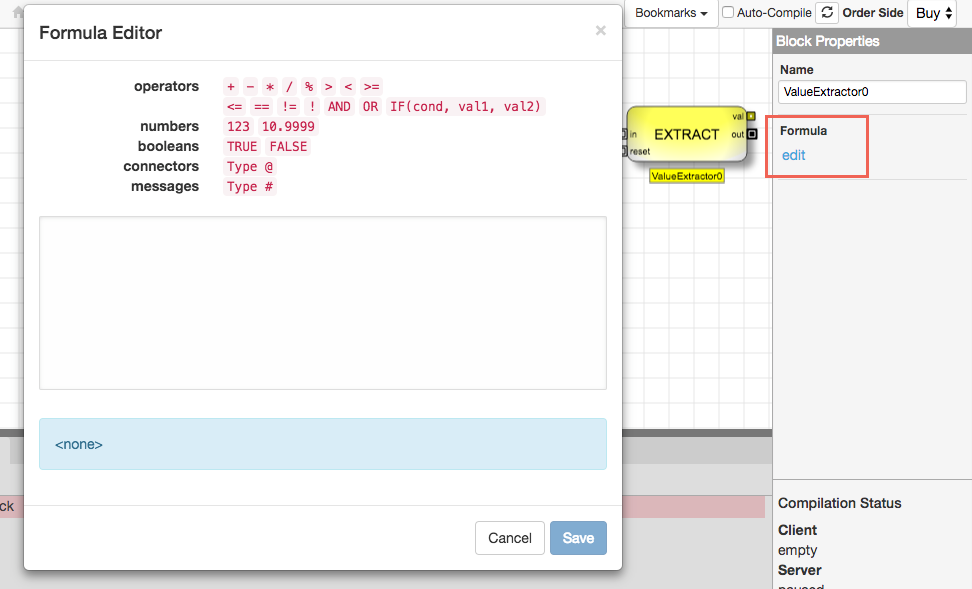The Formula Editor shows the types of values and operations you can use in a formula, including:

• Arithmetic operators (i.e. +, -, %)
• Misc symbols to define the order of operations, such as parentheses
• Comparison operators (i.e. <, >=, ==, !=)
• Logical operators (i.e. IF, AND, OR, !

Note The IF operator is not currently supported in the Alert block.

• Numbers and Boolean values
• Variables, including values from other ADL block output connectors and values from the discrete event message entering the block
• Block connectors let you use the output from another block in a formula.
• Message fields let a formula use information from the incoming discrete event message.

The Formula Editor provides an auto-complete feature that lets you easily refer to information already available in your algo. When type one of the special characters (@ or #), the editor display a list of items that you can insert as variables into the formula. Also, typing characters after the symbol filters the list to items beginning with those characters.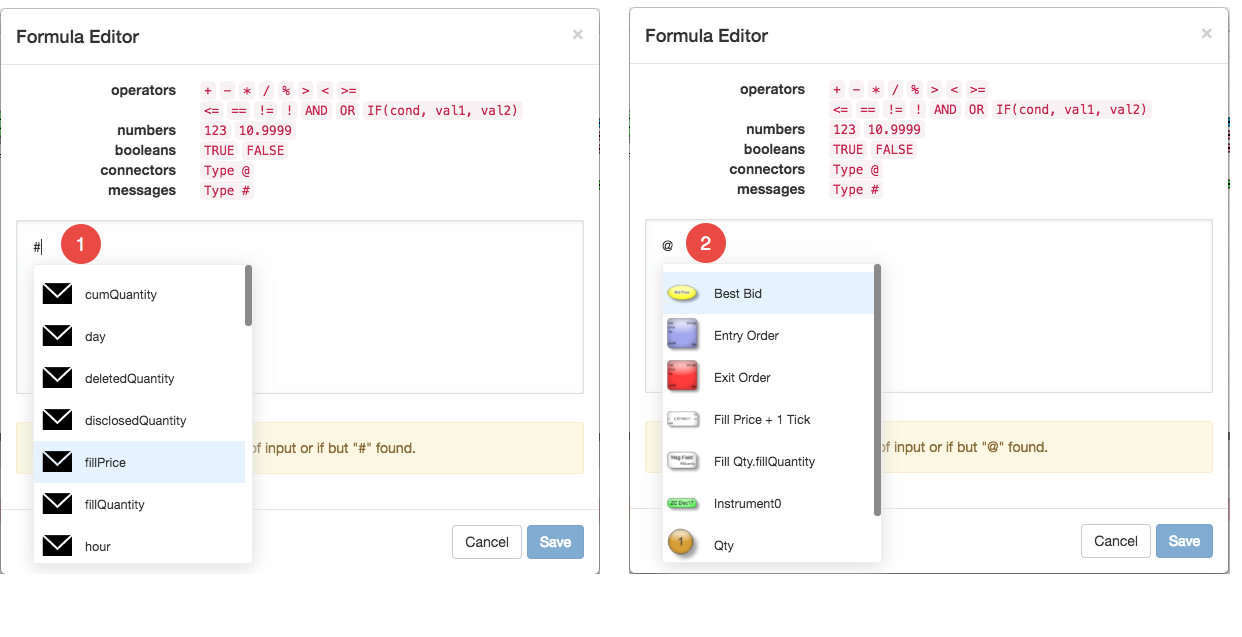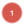Typing # displays a list of all fields available in an an incoming discrete event message.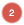Typing @ displays a list of blocks on the canvas that contain one or more continuous output ports.

## Sample formulas

The following samples illustrate some basic formula types.

### Boolean formula

The following formula simply outputs a Boolean value of TRUE, which can then be used by other downstream blocks.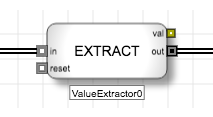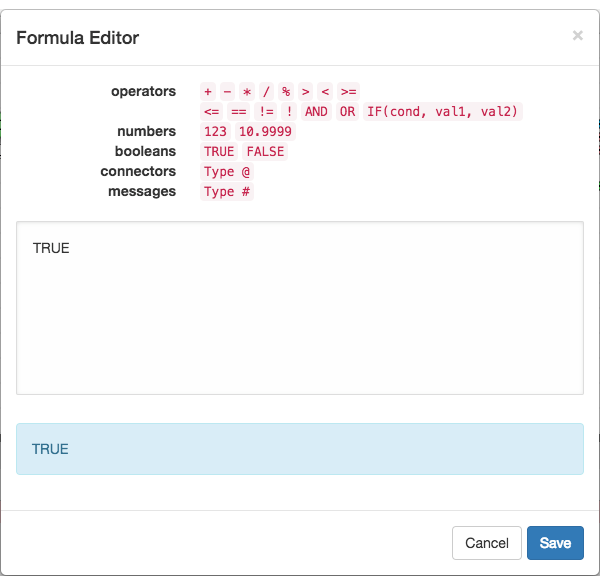### Arithmetic formula

The following formula for the ExitPrice Value Extractor block calculates the price two ticks higher than the fill price, which you could use to submit an exit Sell order two ticks higher than the price of the Buy order fill.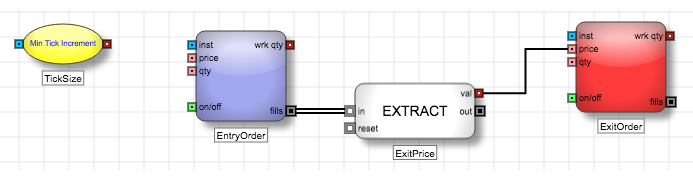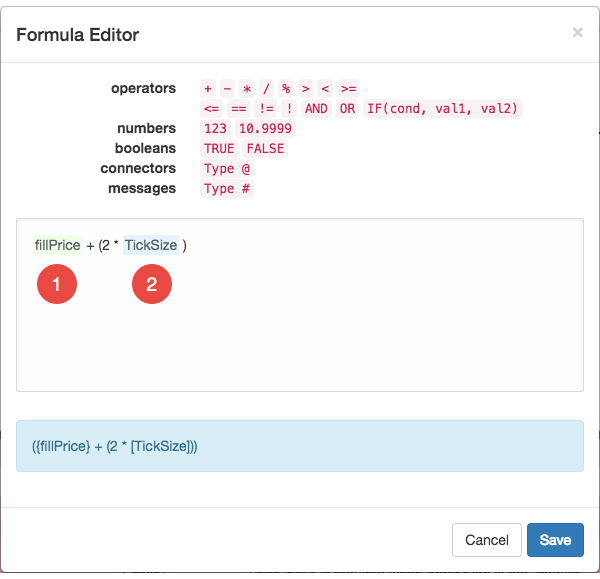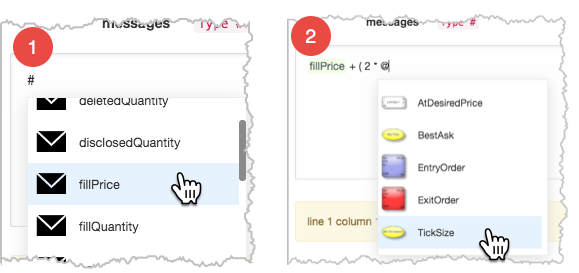To access the value of the fillPrice message field, we type # and select the field.To access the value of the TickSize Field block, we type @ and select the desired block.

### Conditional formula

The following formula outputs a TRUE/FALSE value based on whether the current opposite inside market is two ticks higher than the price of a fill.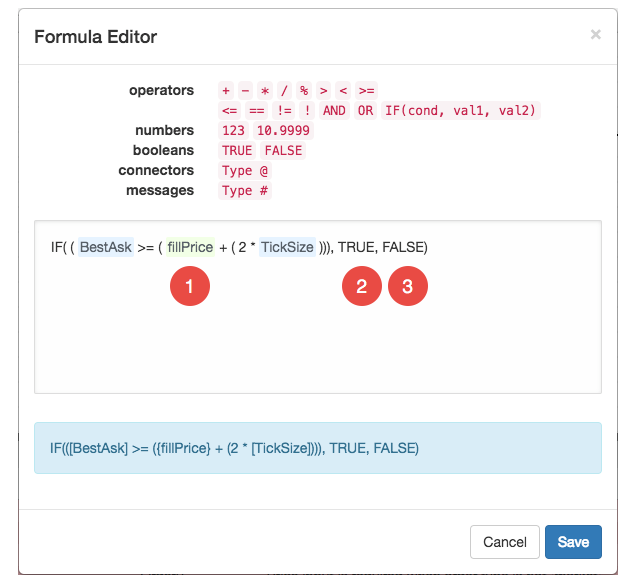1. The IF condition uses the >= operator to compare the value of the BestAsk Field block (the available quantity at the Best Ask price) with the price two ticks away from the fill price (from the previous formula).
2. If the condition is TRUE, the formula outputs the Boolean value TRUE.
3. Otherwise, the formula outputs the Boolean value FALSE.

## Variable fields

The Formula Editor includes drop-down menus that allow you to add values that update dynamically as the algo runs.

• connectors: Lets you reference the value of a continuous output port from any other block in the algorithm. You can also type the connector in the form, [block.connector].

The following connector within the Formula Editor displays the continuous output ports from the Analytics block, named Analytics0 within the algorithm.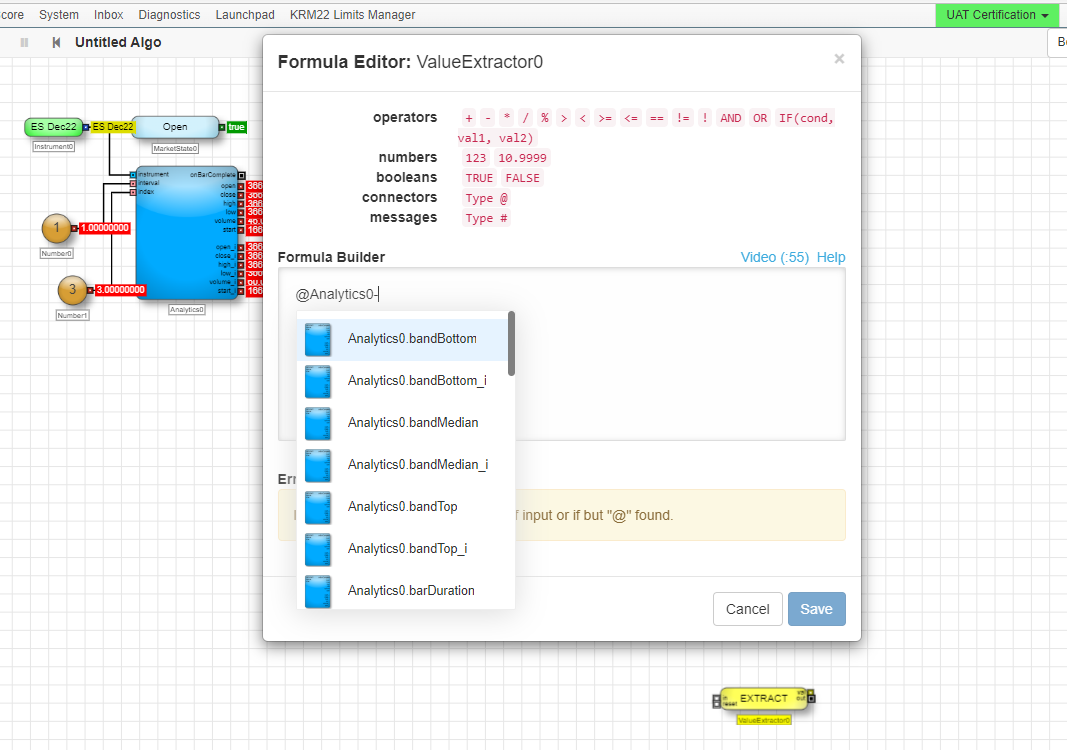Notes:

• Within the example above, you can type @Analytics0, or @Analytics0- (with a dash) to search the output port on a granular level.
• The Formula Editor can only display a maximum of 100 continuous output ports from any other block in the algorithm.
• messages: Lets you extract any of the following information embedded within a discrete event message. You can also type the connector in the form, {fieldName}.

• limitPrice: Price of an new order
• orderQuantity: Total order quantity
• workingQuantity: Working quantity of an order
• fillPrice: Price of a fill
• fillQuantity: Quantity of a fill
• cumQuantity: Sum of fill quantities
• disclosedQuantity: Quantity of a disclosed order visible in the market
• deletedQuantity: Canceled quantity of a deleted order
• stopTrigger: Price at which the respective stop order is activated
• isQuotingOrder: Whether the message was generated by a submitted quote order (valid only for Autospreader order blocks)
• isHedgeOrder: Whether the message was generated by a submitted hedge order (valid only for Autospreader order blocks
• isExternalEvent: Whether the message was generated from a source outside of the algorithm
• isTriggered: Whether an order resulted from a trigger condition
• instrument: Instrument associated with the message
• userFieldN: One of four empty fields that can be populated by a Value Injector and then accessed by a Value Extractor
• "string": (Alert block only) Lets you add customized text to a message string.

## Blocks that use the Formula Editor

The following ADL blocks allow you to create custom formulas.